Example usage of NRSur7dq4 surrogate model.¶

In :
import numpy as np
import matplotlib.pyplot as P
%matplotlib inline

import gwsurrogate
setting __package__ to gwsurrogate.new so relative imports work
__name__ = gwsurrogate.new.spline_evaluation
__package__= gwsurrogate.new
setting __package__ to gwsurrogate.new so relative imports work
setting __package__ to gwsurrogate.new so relative imports work

Download surrogate data, this only needs to be done once¶

In :
# This can take a few minutes
gwsurrogate.catalog.pull('NRSur7dq4')
Out:

Load the surrogate, this only needs to be done once at the start of a script¶

In :

In :
help(sur)
Help on NRSur7dq4 in module gwsurrogate.surrogate object:

class NRSur7dq4(SurrogateEvaluator)
|  A class for the NRSur7dq4 surrogate model presented in Varma et al. 2019,
|  arxiv1905.09300.
|
|  Evaluates gravitational waveforms generated by precessing binary black hole
|  systems with generic mass ratios and spins.
|
|  This model includes the following spin-weighted spherical harmonic modes:
|  2<=ell<=4, -ell<=m<=ell.
|
|  The parameter space of validity is:
|  q \in [1, 6], and |chi1|,|chi2| \in [-1, 1], with generic directions.
|  where q is the mass ratio and chi1/chi2 are the spin vectors of the
|  heavier/lighter BH, respectively.
|
|  The surrogate has been trained in the range
|  q \in [1, 4] and |chi1|/|chi2| \in [-0.8, 0.8], but produces reasonable
|  waveforms in the above range and has been tested against existing
|  NR waveforms in that range.
|
|  See the __call__ method on how to evaluate waveforms.
|  In the __call__ method, x must have format x = [q, chi1, chi2].
|
|  Method resolution order:
|      NRSur7dq4
|      SurrogateEvaluator
|      __builtin__.object
|
|  Methods defined here:
|
|  __init__(self, h5filename)
|
|  ----------------------------------------------------------------------
|  Methods inherited from SurrogateEvaluator:
|
|  __call__(self, q, chiA0, chiB0, M=None, dist_mpc=None, f_low=None, f_ref=None, dt=None, df=None, times=None, freqs=None, mode_list=None, ellMax=None, inclination=None, phi_ref=0, precessing_opts=None, tidal_opts=None, par_dict=None, units='dimensionless', skip_param_checks=False, taper_end_duration=None)
|      INPUT
|      =====
|      q :         Mass ratio, mA/mB >= 1.
|      chiA0:      Dimensionless spin vector of the heavier black hole at
|                  reference epoch.
|      chiB0:      Dimensionless spin vector of the lighter black hole at
|                  reference epoch.
|
|                  This follows the same convention as LAL, where the spin
|                  components are defined as:
|                  \chi_z = \chi \cdot \hat{L}, where L is the orbital angular
|                      momentum vector at the epoch.
|                  \chi_x = \chi \cdot \hat{n}, where n = body2 -> body1 is the
|                      separation vector at the epoch. body1 is the heavier body.
|                  \chi_y = \chi \cdot \hat{L \cross n}.
|                  These spin components are frame-independent as they are
|                  defined using vector inner products. This is equivalent to
|                  specifying the spins in the coorbital frame used in the
|                  surrogate papers.
|
|      M, dist_mpc: Either specify both M and dist_mpc or neither.
|          M        :  Total mass (solar masses). Default: None.
|          dist_mpc :  Distance to binary system (MegaParsecs). Default: None.
|
|      f_low :     Instantaneous initial frequency of the (2, 2) mode. In
|                  practice, this is estimated to be twice the initial orbital
|                  frequency in the coprecessing frame. Note: the coprecessing
|                  frame is the minimal rotation frame of arXiv:1110.2965.
|
|                  f_low should be in cycles/M if units = 'dimensionless',
|                  should be in Hertz if units = 'mks'.
|                  If 0, the entire waveform is returned.
|                  Default: None, must be specified by user.
|
|                  NOTE: For some models like NRSur7dq4, f_low=0 is recommended.
|                  The role of f_low is only to truncate the lower frequencies
|                  before returning the waveform. Since this model is already
|                  very short, this truncation is not required. On the other hand,
|                  f_ref is used to set the reference epoch, and can be freely
|                  specified.
|
|                  WARNING: Using f_low=0 with a small dt (like 0.1M) can lead to
|                  very expensive evaluation for hybridized surrogates like
|                  NRHybSur3dq8.
|
|      f_ref:      Frequency used to set the reference epoch at which the
|                  reference frame is defined and the spins are specified.
|                  See below for definition of the reference frame.
|                  Should be in cycles/M if units = 'dimensionless', should be
|                  in Hertz if units = 'mks'.
|                  Default: If f_ref is not given, we set f_ref = f_low. If
|                  f_low is 0, this corresponds to the initial index.
|
|                  For time domain models, f_ref is used to determine a t_ref,
|                  such that the orbital frequency in the coprecessing frame
|                  equals f_ref/2 at t=t_ref.
|
|      dt, df :    Time/Frequency step size, specify at most one of dt/df,
|                  depending on whether the surrogate is a time/frequency domain
|                  surrogate.
|                  Default: None. If None, the internal domain of the surrogate is
|                  used, which can be nonuniformly sampled.
|                  dt (df) Should be in M (cycles/M) if units = 'dimensionless',
|                  should be in seconds (Hertz) if units = 'mks'. Do not specify
|                  times/freqs if using dt/df.
|
|
|      times, freqs:
|                  Array of time/frequency samples at which to evaluate the
|                  waveform, depending on whether the surrogate is a
|                  time/frequency domain surrogate. time (freqs) should be in
|                  M (cycles/M) if units = 'dimensionless', should be in
|                  seconds (Hertz) if units = 'mks'. Do not specify dt/df if
|                  using times/freqs. Default None.
|
|      ellMax:     Maximum ell index for modes to include. All available m
|                  indicies for each ell will be included automatically.
|                  Default: None, in which case all available modes wll be
|                  included.
|
|      mode_list : A list of (ell, m) modes tuples to be included.
|                  Example: mode_list = [(2,2),(2,1)].
|                  Default: None, in which case all available modes are included.
|                  The m<0 modes will automatically be included for nonprecessing
|                  models. At most one of ellMax and mode_list can be specified.
|
|                  Note: mode_list is allowed only for nonprecessing models; for
|                  precessing models use ellMax. For precessing systems, all m
|                  indices of a given ell index mix with each other, so there is
|                  no clear hierarchy. To get the individual modes just don't
|                  specify inclination and a dictionary of modes will be returned.
|
|      phi_ref :   The angle on the plane of the orbit from the line of ascending
|                  nodes to the position of the body 1 at reference epoch. Can
|                  also be thought of as the orbital phase at reference epoch.
|                  Default: 0.
|
|      inclination : Inclination angle between the orbital angular momentum
|                  direction at the reference epoch and the line-of-sight to the
|                  observer.
|                  If inclination is None, the mode data is returned as a
|                  dictionary.
|                  If specified, the complex strain (h = hplus -i hcross)
|                  evaluated at (inclination, pi/2) on the sky of the reference
|                  frame is returned. This follows the same convention as LAL,
|                  where the azimuthal angle is set through phi_ref. See below
|                  for definition of the reference frame.
|                  Default: None.
|
|      precessing_opts:
|                  A dictionary containing optional parameters for a precessing
|                  surrogate model. Default: None.
|                  Allowed keys are:
|                  quat_ref: The unit quaternion (length 4 vector) giving the
|                      rotation from the coprecessing frame to the inertial frame
|                      at the reference epoch.
|                      Default: None, in which case the spins in the coprecessing
|                      frame are equal to the spins in the inertial frame.
|                  return_dynamics:
|                      Return the frame dynamics and spin evolution along with
|                      the waveform. Default: False.
|                  use_lalsimulation_conventions:
|                      If True, interprets the spin directions and init_orbphase
|                      using lalsimulation conventions. Specifically, before
|                      evaluating the surrogate, the spins will be rotated about
|                      the z-axis by init_phase. Default: True (see
|                      DynamicsSurrogate, which is the only place this option is
|                      used).
|                  Example: precessing_opts = {
|                                      'quat_ref': [1,0,0,0],
|                                      'return_dynamics': True
|                                      }
|
|      tidal_opts:
|                  A dictionary containing optional parameters for a tidal
|                  surrogate model. Default: None.
|                  Allowed keys are:
|                  Lambda1: The tidal deformability parameter for the heavier
|                      object.
|                  Lambda2: The tidal deformability parameter for the lighter
|                      object.
|                  Example: tidal_opts = {'Lambda1': 200, 'Lambda2': 300}
|
|
|      par_dict:   A dictionary containing any additional parameters needed for a
|                  particular surrogate model. Default: None.
|
|      units:      'dimensionless' or 'mks'. Default: 'dimensionless'.
|                  If 'dimensionless': Any of f_low, f_ref, dt, df, times and
|                      freqs, if specified, must be in dimensionless units. That
|                      is, dt/times should be in units of M, while f_ref, f_low
|                      and df/freqs should be in units of cycles/M.
|                      M and dist_mpc must be None. The waveform and domain are
|                      returned as dimensionless quantities as well.
|                  If 'mks': Any of f_low, f_ref, dt, df, times and freqs, if
|                      specified, must be in MKS units. That is, dt/times should
|                      be in seconds, while f_ref, f_low and df/freqs should be
|                      in Hz. M and dist_mpc must be specified. The waveform and
|                      domain are returned in MKS units as well.
|
|
|      skip_param_checks :
|                  Skip sanity checks for inputs. Use this if you want to
|                  extrapolate outside allowed range. Default: False.
|
|      taper_end_durataion:
|                  Taper the last TAPER_END_DURATION (M) of a time-domain waveform
|                  in units of M. For exmple, passing 40 will taper the last 40M.
|                  When set to None, no taper is applied
|                  Default: None.
|
|      RETURNS
|      =====
|
|      domain, h, dynamics
|
|
|      domain :    Array of time/frequency samples corresponding to h and
|                  dynamics, depending on whether the surrogate is a
|                  time/frequency domain model. This is the same as times/freqs
|                  if times/freqs are given as an inputs.
|                  For time domain models the time is set to 0 at the peak of
|                  the waveform. The time (frequency) values are in M (cycles/M)
|                  if units = 'dimensionless', they are in seconds (Hertz) if
|                  units = 'mks'
|
|      h :         The waveform.
|                      If inclination is specified, the complex strain (h = hplus
|                      -i hcross) evaluated at (inclination, pi/2) on the sky of
|                      the reference frame is returned. This follows the LAL
|                      convention, see below for details.  This includes all modes
|                      given in the ellMax/mode_list argument. For nonprecessing
|                      systems the m<0 modes are automatically deduced from the
|                      m>0 modes. To see if a model is precessing check
|                      self.keywords.
|
|                      Else, h is a dictionary of available modes with (l, m)
|                      tuples as keys. For example, h22 = h[(2,2)].
|
|                      If M and dist_mpc are given, the physical waveform
|                      at that distance is returned. Else, it is returned in
|                      code units: r*h/M extrapolated to future null-infinity.
|
|      dynamics:   A dict containing the frame dynamics and spin evolution. This
|                  is None for nonprecessing models. This is also None if
|                  return_dynamics in precessing_opts is False (Default).
|
|                  The dynamics include (L=len(domain)):
|
|                  q_copr = dynamics['q_copr']
|                      The quaternion representing the coprecessing frame with
|                      shape (4, L)
|                  orbphase = dynamics['orbphase']
|                      The orbital phase in the coprecessing frame with length L.
|                  chiA = dynamics['chiA']
|                      The inertial frame chiA with shape (L, 3)
|                  chiB = dynamics['chiB']
|                      The inertial frame chiB with shape (L, 3)
|
|
|      IMPORTANT NOTES:
|      ===============
|
|      The reference frame (or inertial frame) is defined as follows:
|          The +ve z-axis is along the orbital angular momentum at the reference
|          epoch. The orbital phase at the reference epoch is phi_ref. This means
|          that the separation vector from the lighter BH to the heavier BH is at
|          an azimuthal angle phi_ref from the +ve x-axis, in the orbital plane at
|          the reference epoch. The y-axis completes the right-handed triad. The
|          reference epoch is set using f_ref.
|
|          Now, if inclination is given, the waveform is evaluated at
|          (inclination, pi/2) in the reference frame. This agrees with the LAL
|          convention. See LIGO DCC document T1800226 for the LAL frame diagram.
|
|  ----------------------------------------------------------------------
|  Data descriptors inherited from SurrogateEvaluator:
|
|  __dict__
|      dictionary for instance variables (if defined)
|
|  __weakref__
|      list of weak references to the object (if defined)

Evaluate the waveform¶

Evaluate waveform modes in dimensionless units (default)¶

In :
q = 4
chiA = [-0.2, 0.4, 0.1]
chiB = [-0.5, 0.2, -0.4]
dt = 0.1        # timestep size, Units of M
f_low = 0       # initial frequency, f_low=0 returns the full surrogate
t, h, dyn = sur(q, chiA, chiB, dt=dt, f_low=f_low)   # dyn stands for dynamics, do dyn.keys() to see contents
In :
# Let's see all available modes
print( sorted(h.keys()) )
[(2, -2), (2, -1), (2, 0), (2, 1), (2, 2), (3, -3), (3, -2), (3, -1), (3, 0), (3, 1), (3, 2), (3, 3), (4, -4), (4, -3), (4, -2), (4, -1), (4, 0), (4, 1), (4, 2), (4, 3), (4, 4)]
In :
P.plot(t, h[(2,2)].real, label='l2m2 real')
P.plot(t, h[(2,1)].real, label='l2m1 real')
P.plot(t, h[(3,3)].real, label='l3m3 real')
P.ylabel('Re[$h_{lm}$]', fontsize=18)
P.xlabel('t [M]', fontsize=18)
P.legend()
Out:
<matplotlib.legend.Legend at 0x1a1d51f150>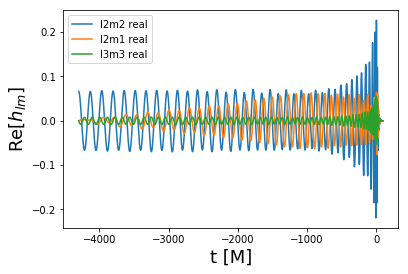Evaluate waveform modes in physical units¶

In :
q = 4
chiA = [-0.2, 0.4, 0.1]
chiB = [-0.5, 0.2, -0.4]
f_ref = 20         # Reference frequecny in Hz. The spins are assumed to specified at this frequency
f_low = 0          # initial frequency, f_low=0 returns the full surrogate
M = 70             # Total masss in solar masses
dist_mpc = 100     # distance in megaparsecs
dt = 1./4096       # step size in seconds
ellMax = 4         # Highest ell index for modes to use

# dyn stands for dynamics, do dyn.keys() to see contents
t, h, dyn = sur(q, chiA, chiB, dt=dt, f_low=f_low, f_ref=f_ref, ellMax=ellMax, M=M, dist_mpc=dist_mpc, units='mks')

P.plot(t, h[(2,2)].real, label='l2m2 real')
P.plot(t, h[(2,1)].real, label='l2m1 real')
P.ylabel('Re[$h_{lm}$]', fontsize=18)
P.xlabel('t [s]', fontsize=18)
P.legend()
Out:
<matplotlib.legend.Legend at 0x1a1d1a78d0>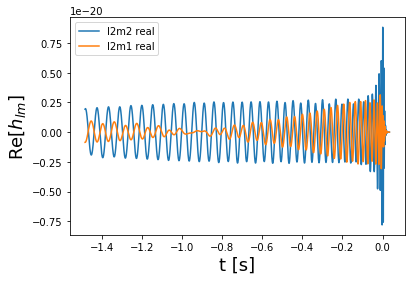Evaluate waveform at a point on the sky¶

In :
q = 4
chiA = [-0.2, 0.4, 0.1]
chiB = [-0.5, 0.2, -0.4]
f_ref = 20         # Reference frequecny in Hz. The spins are assumed to specified at this frequency
f_low = 0          # initial frequency, f_low=0 returns the full surrogate
M = 70             # Total masss in solar masses
dist_mpc = 100     # distance in megaparsecs
dt = 1./4096       # step size in seconds
ellMax = 4         # Highest ell index for modes to use
inclination = np.pi/4
phi_ref = np.pi/5

# Will only include modes ell<=ellMax
# Returns h = h_+ -i h_x at (inclination, phi_ref) in the sky of the source frame
# dyn stands for dynamics, do dyn.keys() to see contents
t, h, dyn = sur(q, chiA, chiB, dt=dt, f_low=f_low, f_ref=f_ref, ellMax=ellMax, M=M, dist_mpc=dist_mpc,
inclination=inclination, phi_ref=phi_ref, units='mks')

P.plot(t, h.real)
P.ylabel('$h_{+}$ $(\iota, \phi_{ref})$', fontsize=18)
P.xlabel('t [s]', fontsize=18)
Out:
Text(0.5,0,'t [s]')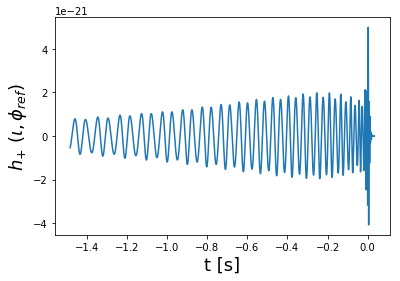Dynamics Surrogate¶

In :
q = 4
chiA = [-0.2, 0.4, 0.1]  # unless f_ref is given the spins are assumed to be given at the start of the waveform
chiB = [-0.5, 0.2, -0.4]
dt = 0.1        # step size, Units of M
f_low = 0       # initial frequency, f_low=0 returns the full surrogate
t, h, dyn = sur(q, chiA, chiB, dt=dt, f_low=f_low, precessing_opts={'return_dynamics': True})        # dyn stands for dynamics, do dyn.keys() to see contents
In :
# See all available dynamics data
dyn.keys()
Out:
['chiB', 'orbphase', 'chiA', 'q_copr']
In :
P.figure(1)
P.plot(t, dyn['chiA'][:,0], label='$\chi_{Ax}$')
P.plot(t, dyn['chiA'][:,1], label='$\chi_{Ay}$')
P.plot(t, dyn['chiA'][:,2], label='$\chi_{Az}$')
P.ylabel('$\chi_A$', fontsize=18)
P.xlabel('t', fontsize=18)
P.title('Spin of heavier BH')
P.legend(fontsize=14)

P.figure(2)
P.plot(t, dyn['chiB'][:,0], label='$\chi_{Bx}$')
P.plot(t, dyn['chiB'][:,1], label='$\chi_{By}$')
P.plot(t, dyn['chiB'][:,2], label='$\chi_{Bz}$')
P.ylabel('$\chi_B$', fontsize=18)
P.xlabel('t', fontsize=18)
P.title('Spin of lighter BH')
P.legend(fontsize=14)

P.figure(3)
P.plot(t, dyn['orbphase'][:,])
P.ylabel('$\phi_{\mathrm{orb}}$', fontsize=18)
P.xlabel('t', fontsize=18)
P.title('Orbital phase')

P.figure(4)
P.plot(t, dyn['q_copr'][0,:], label='$\hat{Q}_0$')
P.plot(t, dyn['q_copr'][1,:], label='$\hat{Q}_1$')
P.plot(t, dyn['q_copr'][2,:], label='$\hat{Q}_2$')
P.plot(t, dyn['q_copr'][3,:], label='$\hat{Q}_3$')
P.ylabel('$\hat{Q}$', fontsize=18)
P.xlabel('t', fontsize=18)
P.title('Coprecessing frame quaternions')
P.legend(fontsize=14)
Out:
<matplotlib.legend.Legend at 0x1a1e358dd0>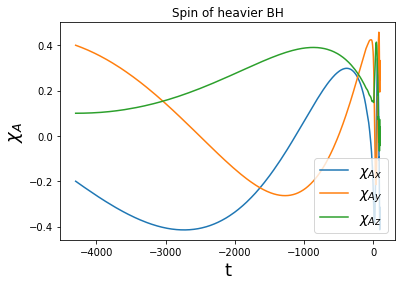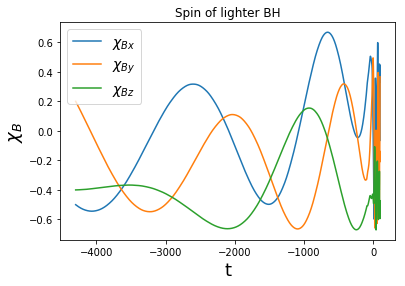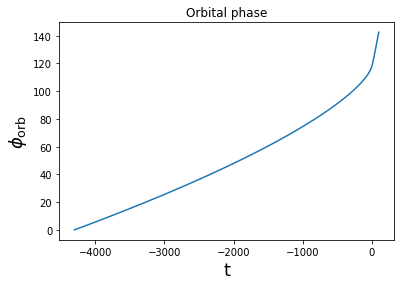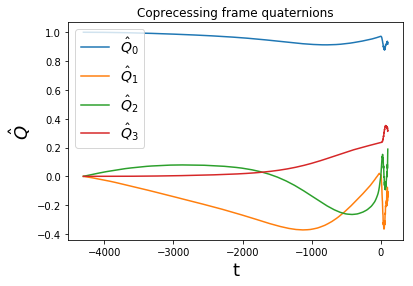In [ ]: